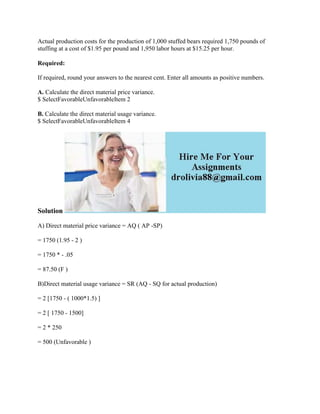Seu SlideShare está sendo baixado. ×

# Actual production costs for the production of 1-000 stuffed bears requ.docx

Anúncio
Anúncio
Anúncio
Anúncio
Anúncio
Anúncio
Anúncio
Anúncio
Anúncio
Anúncio
AnúncioCarregando em…3
×

1 de 2 Anúncio

# Actual production costs for the production of 1-000 stuffed bears requ.docx

Actual production costs for the production of 1,000 stuffed bears required 1,750 pounds of stuffing at a cost of \$1.95 per pound and 1,950 labor hours at \$15.25 per hour.
Required:
If required, round your answers to the nearest cent. Enter all amounts as positive numbers.
A. Calculate the direct material price variance.
\$ SelectFavorableUnfavorableItem 2
B. Calculate the direct material usage variance.
\$ SelectFavorableUnfavorableItem 4
Solution
A) Direct material price variance = AQ ( AP -SP)
= 1750 (1.95 - 2 )
= 1750 * - .05
= 87.50 (F )
B)Direct material usage variance = SR (AQ - SQ for actual production)
= 2 [1750 - ( 1000*1.5) ]
= 2 [ 1750 - 1500]
= 2 * 250
= 500 (Unfavorable )
.

Actual production costs for the production of 1,000 stuffed bears required 1,750 pounds of stuffing at a cost of \$1.95 per pound and 1,950 labor hours at \$15.25 per hour.
Required:
If required, round your answers to the nearest cent. Enter all amounts as positive numbers.
A. Calculate the direct material price variance.
\$ SelectFavorableUnfavorableItem 2
B. Calculate the direct material usage variance.
\$ SelectFavorableUnfavorableItem 4
Solution
A) Direct material price variance = AQ ( AP -SP)
= 1750 (1.95 - 2 )
= 1750 * - .05
= 87.50 (F )
B)Direct material usage variance = SR (AQ - SQ for actual production)
= 2 [1750 - ( 1000*1.5) ]
= 2 [ 1750 - 1500]
= 2 * 250
= 500 (Unfavorable )
.

Anúncio
Anúncio

### Actual production costs for the production of 1-000 stuffed bears requ.docx

1. 1. Actual production costs for the production of 1,000 stuffed bears required 1,750 pounds of stuffing at a cost of \$1.95 per pound and 1,950 labor hours at \$15.25 per hour. Required: If required, round your answers to the nearest cent. Enter all amounts as positive numbers. A. Calculate the direct material price variance. \$ SelectFavorableUnfavorableItem 2 B. Calculate the direct material usage variance. \$ SelectFavorableUnfavorableItem 4 Solution A) Direct material price variance = AQ ( AP -SP) = 1750 (1.95 - 2 ) = 1750 * - .05 = 87.50 (F ) B)Direct material usage variance = SR (AQ - SQ for actual production) = 2 [1750 - ( 1000*1.5) ] = 2 [ 1750 - 1500] = 2 * 250 = 500 (Unfavorable )